Ask your WordPress questions! Pay money and get answers fast!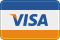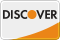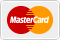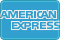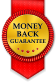# Create array of hours within wordpress loop WordPress

• SOLVED

I have a wordpress loop and need to create an array of the hours within each day, this works fine as long as the start and end do not span multiple days. Eg: 11pm to 7am the following day. I would like to create a new array item for each 24 hour period. SO if a date spans from one day to the next I would rather have multiple dates.

``` if ( \$the_query->have_posts() ) { while ( \$the_query->have_posts() ) { \$the_query->the_post(); \$meta = get_post_meta(\$the_query->post->ID); \$start = date('G',strtotime(\$meta['start'])); \$end = date('G',strtotime(\$meta['end'])); \$arr[date('Y/m/d',strtotime(get_the_date()))] = array( 'start' => \$start, 'end' => \$end ); } } ```

array looks like this,

``` Array ( [2021/10/28] => Array ( [start] => 0 [end] => 8 ) [2021/10/27] => Array ( [start] => 0 [end] => 8 ) [2021/10/25] => Array ( [start] => 0 [end] => 8 ) [2021/10/22] => Array ( [start] => 0 [end] => 8 ) [2021/10/21] => Array ( [start] => 0 [end] => 8 ) [2021/10/20] => Array ( [start] => 0 [end] => 8 ) [2021/10/19] => Array ( [start] => 0 [end] => 8 ) ) ```

2021-10-28

can you share the desired output of the array when date date spans from one day to the next ?

see the array under the code sample

if it spanned two days would like like this,

``` [2021/10/20] => Array ( [start] => 0 [end] => 8 ) [2021/10/19] => Array ( [start] => 16 [end] => 23 ) ```

how you have added the start and end date to the post?
Are you using any plugin ? Tribe events?

no its meta data with the start and end date. each post has one start and end date.

they are just posts why would you ever need a video ? I have posts and they contain dates sometimes the dates span past one day. I need to correlate the dates so that it breaks up the hours over multiple days in the array.

2021-10-28

To get the desired array, firstly, we need to arrive at the difference between the start and end dates. And then, loop over the number of days between start and end dates with few conditions that fits our requirement and finally feed them into the array.

Here is a code you can try:
``` if ( \$the_query->have_posts() ) { while ( \$the_query->have_posts() ) { \$the_query->the_post(); \$meta = get_post_meta(\$the_query->post->ID); \$start = date('G',strtotime(\$meta['start'])); \$end = date('G',strtotime(\$meta['end'])); \$start_datetime = strtotime(\$meta['start']); \$end_datetime = strtotime(\$meta['end']); \$start_date = date('Y/m/d',\$start_datetime); \$end_date = date('Y/m/d',\$end_datetime); // Calculating the difference in timestamps \$diff = \$end_datetime - \$start_datetime; // 1 day = 24 hours // 24 * 60 * 60 = 86400 seconds \$daysbetween = (abs(round(\$diff / 86400)) +1); // echo \$daysbetween; for (\$i = 1; \$i <= \$daysbetween; \$i++) { if ((\$i == 1 ) and (\$i == \$daysbetween)) { // single date \$datekey = \$start_date; \$startval = \$start; \$endval = \$end; } if ((\$i == 1 ) and (\$i != \$daysbetween)) { // more days exist, this is first date \$datekey = \$start_date; \$startval = \$start; \$endval = '23'; } if ((\$i > 1) and (\$i < \$daysbetween)) { // days in between \$datekey = date('Y/m/d', strtotime(\$start_date .'+' .(\$i-1) .' days')); \$startval = '0'; \$endval = '23'; } if ((\$i > 1) and (\$i == \$daysbetween)) { // the last date \$datekey = \$end_date; \$startval = '0'; \$endval = \$end; } //push the item into your array \$arr[\$datekey] = array( 'start' => \$startval, 'end' => \$endval ); } } } ```

your array will be in \$arr. You can print it or use it further in your application. The output will be in this format that you expected:

``` Array ( [2014/08/12] => Array ( [start] => 9 [end] => 23 ) [2014/08/13] => Array ( [start] => 0 [end] => 11 ) ) ```

Here is a link where you can view the output of this code (slightly modified to remove wordpress functions):
https://paiza.io/projects/e/Oz6G6M28DUjOM0Mf1ozccA

this works great however sometimes I get results with a 0 as the endval and something like 16 as the startval. Sometimes I also get 0 for both; would want anything 0,0 to be gone.

it would be better that if the dates are within these blocks eg:

0-8
8-16
16-23

That if just be these rather than the specific dates, this is for shift evaluation.

what happens is we have one person come in for a short shift like 2 hours just to cover and it messes things up. I think the best would be if they have a shift that cover over 2 blocks eg:

12pm - 6pm they would get blocks

8-16
16-23

as they worked within these periods.

in your sample code if I run eg:

\$meta = array(
'start'=>array('2021-10-28 4:00:00pm'),
'end' =>array('2021-10-29 12:00:00am')
);

or this; however in most cases this works great.
``` \$meta = array( 'start'=>array('2021-10-28 16:00:00'), 'end' =>array('2021-10-29 00:00:00') ); ```

Glad to know the code works in most cases.

Now, regarding shifts how do you expect the output to be? Can you provide a sample array output with shifts as blocks?

Are you expecting this to be:

Shifts Option 1:
``` Array ( [2014/08/12-ShiftA] => Array ( [start] => 6 [end] => 7 ) [2014/08/12-ShiftB] => Array ( [start] => 8 [end] => 10 ) [2014/08/13-ShiftA] => Array ( [start] => 0 [end] => 6 ) ) ```

or Shifts Option 2:
``` Array ( [2014/08/12] => Array ( [0-8] => Array ( [start] => 6 [end] => 7 ) [8-16] => Array ( [start] => 8 [end] => 10 ) ) [2014/08/13] => Array ( [0-8] => Array ( [start] => 4 [end] => 6 ) ) ) ```

Either way or any other expected output you have in mind, I request you to kindly increase the budget for this question or have it as a separate question because I believe this is an extension of the scope of the original question. I am sure you'll agree that the original question has been answered to a great extent. Thanks for understanding.